JEE  >  VITEEE Maths Test - 1

# VITEEE Maths Test - 1

Test Description

## 40 Questions MCQ Test VITEEE: Subject Wise and Full Length MOCK Tests | VITEEE Maths Test - 1

VITEEE Maths Test - 1 for JEE 2023 is part of VITEEE: Subject Wise and Full Length MOCK Tests preparation. The VITEEE Maths Test - 1 questions and answers have been prepared according to the JEE exam syllabus.The VITEEE Maths Test - 1 MCQs are made for JEE 2023 Exam. Find important definitions, questions, notes, meanings, examples, exercises, MCQs and online tests for VITEEE Maths Test - 1 below.
Solutions of VITEEE Maths Test - 1 questions in English are available as part of our VITEEE: Subject Wise and Full Length MOCK Tests for JEE & VITEEE Maths Test - 1 solutions in Hindi for VITEEE: Subject Wise and Full Length MOCK Tests course. Download more important topics, notes, lectures and mock test series for JEE Exam by signing up for free. Attempt VITEEE Maths Test - 1 | 40 questions in 50 minutes | Mock test for JEE preparation | Free important questions MCQ to study VITEEE: Subject Wise and Full Length MOCK Tests for JEE Exam | Download free PDF with solutions
 1 Crore+ students have signed up on EduRev. Have you?
VITEEE Maths Test - 1 - Question 1

### The maximum value of logx/x in (2, ∞) is

Detailed Solution for VITEEE Maths Test - 1 - Question 1
1/e
let f(x)=logx/x
to get max of f(x)... fi(x)=0 u get some value of x
substitute it in f(x) u get max value of f(x)
VITEEE Maths Test - 1 - Question 2

### The area enclosed by the parabola y2 = 8x and the line y = 2x is

VITEEE Maths Test - 1 - Question 3

### Locus of a point P equidistant from two fixed points A and B is ____________

Detailed Solution for VITEEE Maths Test - 1 - Question 3

The line which makes equal distance from the two fixed points will definitely pass through the midpoint of line joining the two points and will definitely perpendicular to the line formed by joining the two points.

VITEEE Maths Test - 1 - Question 4

The real part of 1/(1-cosθ + i sin θ) is

VITEEE Maths Test - 1 - Question 5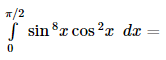VITEEE Maths Test - 1 - Question 6

If the matrix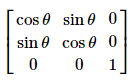is singular, then θ =

Detailed Solution for VITEEE Maths Test - 1 - Question 6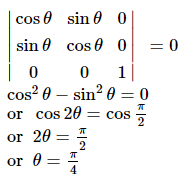VITEEE Maths Test - 1 - Question 7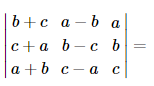VITEEE Maths Test - 1 - Question 8

If f x = x 2 | x | for x ≠ 0, f 0 = 0, then f x at x = 0 is

VITEEE Maths Test - 1 - Question 9

If f( x)   = sin π [ x ] then f ′ (1 − 0)    is equal to

Detailed Solution for VITEEE Maths Test - 1 - Question 9

Solution :- f′(1-0)= lim h→0 f(1-0-h)−f(1-0)

= lim h→0 (sinπ[1-0-h]−sinπ[1-0])/h

= lim h→0 (sinπ−sinπ)/h=0

VITEEE Maths Test - 1 - Question 10

The family of curves, in which the subtangent at any point to any curve is double the abscissa, is given by

VITEEE Maths Test - 1 - Question 11

Observe the following statements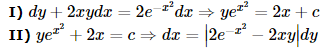Which of the following is a correct statement?

VITEEE Maths Test - 1 - Question 12

The solution of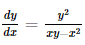is

VITEEE Maths Test - 1 - Question 13

The solution of the equation (dy/dx)=cotx coty is

Detailed Solution for VITEEE Maths Test - 1 - Question 13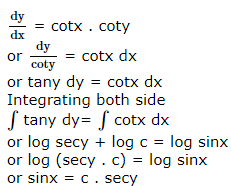VITEEE Maths Test - 1 - Question 14

The differential of sin-1[(1-x)/(1+x)] w.r.t. √x is equal to

VITEEE Maths Test - 1 - Question 15

Suppose that g(x) = 1 + √x and f(g(x)) = 3 + 2√x + x, then f(x) is

VITEEE Maths Test - 1 - Question 16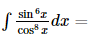VITEEE Maths Test - 1 - Question 17

∫[(1)/(1-x2)]dx=

VITEEE Maths Test - 1 - Question 18

If sin-1x + sin-1y = 2π/3, then cos-1 x + cos-1 y is equal to

VITEEE Maths Test - 1 - Question 19

if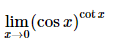is

Detailed Solution for VITEEE Maths Test - 1 - Question 19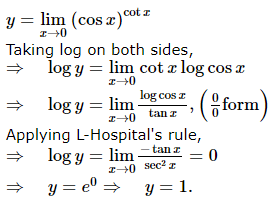VITEEE Maths Test - 1 - Question 20
If p : A man is happy
q : A man is rich
Then , the statement , ' if a man is not happy , then he is not rich ' is written as
VITEEE Maths Test - 1 - Question 21

If f : ℝ → ℝ is defined by f x =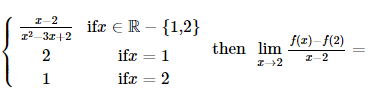VITEEE Maths Test - 1 - Question 22

If B is a non-singular matrix and A is a square matrix, then det (B-1AB) =

VITEEE Maths Test - 1 - Question 23

m [ − 3 4 ] + n [ 4 − 3 ] = [ 10 − 11 ]

so, 3 m + 7 n =

Detailed Solution for VITEEE Maths Test - 1 - Question 23

m[ -3 4 ] + n[ 4 -3 ] = [ -3m 4m ] + [ 4n -3n ]

= [ (-3m)+4n 4m+(-3n) ] = [ 10 -11 ]

• we can compare both sides,

-3m + 4n = 10 .... (1)

And 4m - 3n = -11 .... (2)

• Solving both equations

multiply equation (1) by 4 and equation (2) by 3

-12m + 14n = 40

and 12m - 9n = -33

• Add equation (1) and (2)

-12m + 14n + 12m - 9n = 40 - 33

5n = 7

n = 7/5

• Substitute value of n in equation (1)

-3m + 4×(7/5) = 10

-3m + 28/5 = 10

-15m + 28 = 50

-15m = 50 - 28

m = -22/15

• Substitute value of m and n in 3m + 7n

3(-22/15) + 7(7/5) = -22/5 + 49/5

= 27/5

• Hence, value of 3m + 7n is 27/5

VITEEE Maths Test - 1 - Question 24

If the probability that A and B will die within a year are p and q respectively, then the probability that only one of them will be alive at the end of the year, is

VITEEE Maths Test - 1 - Question 25

We have (n+1) white balls and (n+1) black balls. In each set the balls are numbered from 1 to (n+1). If these balls are to be arranged in a row so that two consecutive balls are of different colours, then the number of these arrangements is

Detailed Solution for VITEEE Maths Test - 1 - Question 25

Between (n + 1) white balls there are (n + 2) gaps in which (n + 1) black ball are to arranged.
No. of reqd arrangements = (n + 1)! (n + 1)! = [(n + 1)!]2
Now between (n + 1) black balls (n + 1) white balls are to be filled no. of ways
= (n + 1)! (n + 1)!
∴ Reqd ways = 2[(n + 1)!]2

VITEEE Maths Test - 1 - Question 26

A bag contains 6 white and 4 black balls. Two balls are drawn at random. The probability that they are of the same colour is

VITEEE Maths Test - 1 - Question 27

A fair dice is tossed repeatedly until six shows up 3 times. The probability that exactly 5 tosses are needed is

VITEEE Maths Test - 1 - Question 28

If in ∆ABC ∠A=45o, ∠C=60o, then a+c√2=

VITEEE Maths Test - 1 - Question 29

Let the harmonic means and the geometric mean of two positive numbers be in the ratio 4 : 5. The two numbers are in the ratio

VITEEE Maths Test - 1 - Question 30

Which one of the following is a source of data for primary investigations?

VITEEE Maths Test - 1 - Question 31

If each observation of a raw data, whose variance is σ2, is multiplied by λ, then the variance of new set is

VITEEE Maths Test - 1 - Question 32

The degree of the equation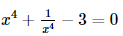is ____

Detailed Solution for VITEEE Maths Test - 1 - Question 32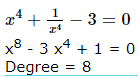VITEEE Maths Test - 1 - Question 33

If the roots of the equation (a2 + b2)x2 - 2(ac + bd)x + (c2 + d2) = 0 are equal is

Detailed Solution for VITEEE Maths Test - 1 - Question 33

(a2 + b2)x2 - 2 (ac + bd)x + (c2 + d2) = 0
For equal root, Discriminant (D) = 0
or [- 2 (ac + bd)]2 - 4.(a2 + b2) (c2 + d2) = 0
or 4 (ac + bd)2 - 4 (a2 + b2) (c2 + d2) = 0
or a2c2 + b2d2 + 2acbd - (a2 + b2) (c2 + d2) = 0
or a2c2 + b2d2 + 2acbd - a2c2 - a2d2 - b2d2
or 2acbd - a2d2 - b2c2 = 0
or (ad - bc)2 = 0

VITEEE Maths Test - 1 - Question 34

If A (1,2,3),B(-1,-1,-1) be the pts., then the distance AB is

VITEEE Maths Test - 1 - Question 35

The plane of intersection of x2+y2+z2+2x+2y+2z+2=0 and 4x2+4y2+4z2+4x+4y+4z-1=0 is

VITEEE Maths Test - 1 - Question 36

The equation 3sin2x + 10cosx - 6 = 0 is satisfied if

VITEEE Maths Test - 1 - Question 37

The angle between the two straight lines represented by 6y2 - xy - x2 + 30y + 36 = 0 is

VITEEE Maths Test - 1 - Question 38

The length of the intercept made by the circle x2 + y2 + 10x - 6y + 9 = 0 on the X-axis is

VITEEE Maths Test - 1 - Question 39

If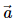makes an acute angle with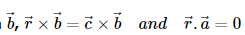, then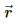is equal to

VITEEE Maths Test - 1 - Question 40

If |a+b|=|a-b|, then the angle between a and b is

## VITEEE: Subject Wise and Full Length MOCK Tests

1 videos|3 docs|73 tests
 Use Code STAYHOME200 and get INR 200 additional OFF Use Coupon Code
Information about VITEEE Maths Test - 1 Page
In this test you can find the Exam questions for VITEEE Maths Test - 1 solved & explained in the simplest way possible. Besides giving Questions and answers for VITEEE Maths Test - 1, EduRev gives you an ample number of Online tests for practice

## VITEEE: Subject Wise and Full Length MOCK Tests

1 videos|3 docs|73 tests